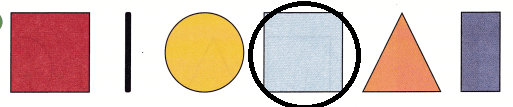# McGraw Hill Math Grade 1 Chapter 11 Lesson 2 Answer Key Shapes Can Be Different Colors

All the solutions provided in McGraw Hill Math Grade 1 Answer Key PDF Chapter 11 Lesson 2 Shapes Can Be Different Colors as per the latest syllabus guidelines.

## McGraw-Hill Math Grade 1 Answer Key Chapter 11 Lesson 2 Shapes Can Be Different Colors

Identify

Circle the matching shapes.

Question 1.Explanation:
The given shape is a circle so, circled the circle shape.
A circle has curved surface around a center point.

Question 2.Explanation:
The given shape is a rectangle so, circled the shape rectangle.
A rectangle has 4 sides and 4 corners, opposite sides are equal.

Question 3.